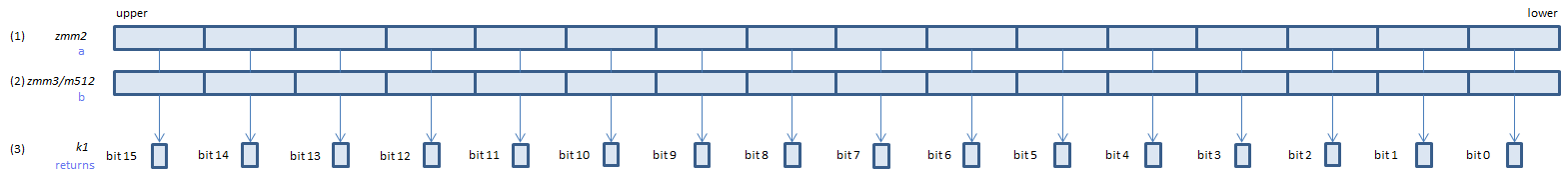﻿ vcmpgeps

## VCMPGEPS - CoMPare Greater Equal Packed Single

VCMPGEPS xmm1, xmm2, xmm3/m128    (V1
__m128 _mm_cmpge_ps(__m128 a, __m128 b)    (S1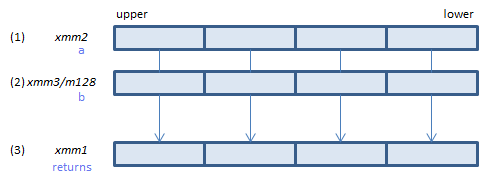For each float, if (1) >= (2) set 1, else set 0, to all bits of the corresponding float of (3).
VCMPGEPS ymm1, ymm2, ymm3/m256    (V1
__m256 _mm256_cmp_ps(__m256 a, __m256 b, _CMP_GE_OS)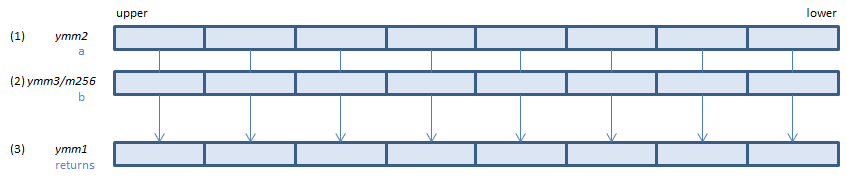For each float, if (1) >= (2) set 1, else set 0, to all bits of the corresponding float of (3).
VCMPGEPS k1{k2}, xmm2, xmm3/m128/m32bcst    (V5+VL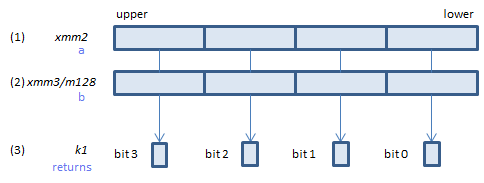For each float, if (1) >= (2) set 1, else set 0, to the corresponding bit of (3).
If k2 bit is 0, the comparison is not done and the corresponding bit of (3) is set to zero. Upper bits of (3) are zero cleared.
VCMPGEPS k1{k2}, ymm2, ymm3/m256/m32bcst    (V5+VL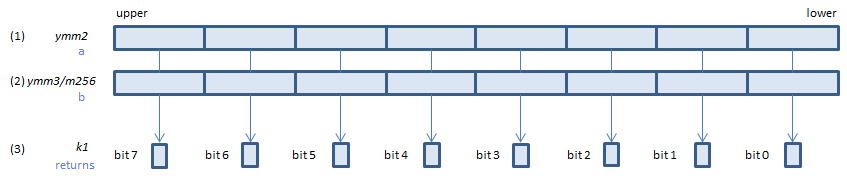For each float, if (1) >= (2) set 1, else set 0, to the corresponding bit of (3).
If k2 bit is 0, the comparison is not done and the corresponding bit of (3) is set to zero. Upper bits of (3) are zero cleared.
VCMPGEPS k1{k2}, zmm2, zmm3/m512/m32bcst{sae}    (V5